### AME 301: Differential Equations, Vibrations and Control Homework 2

 B. GoodwineJ. Lucey Fall, 2003 Issued: September 3, 2003 Due: September 10, 2003

Unless otherwise indicated, all the course text, Boyce and DiPrima, Elementary Differential Equations (and Boundary Value Problems). Each problem is worth 10 points.

Unless otherwise indicated, if a problem calls for a plot of a solution, you can either plot the solution by hand, or use a computer package such as Matlab.

1. Section 3.1: 26
2. Section 3.2:
1. 4
2. 6
3. 9
4. 10
5. 13
6. 27

3. Section 3.4:
1. 11
2. 18
3. 19
4. 26
4. Consider the torsional system illustrated in the following figure.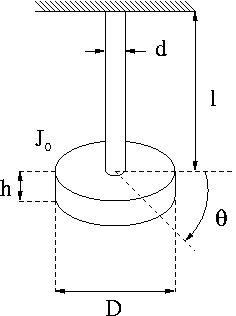From solid mechanics, we know that twisting the shaft by an amount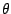requires a torque given by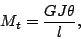where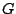is the shear modulus,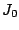is the mass moment of inertia of the disk,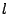is the length of the shaft,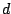is the diameter of the shaft and the polar (area) moment of inertia of the shaft is given by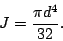1. Determine the equation of motion for this system.
2. Determine the natural frequency.

5. Consider the mass-spring-damper system illustrated in the following figure.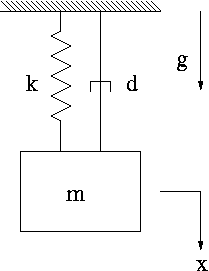1. Determine the equation of motion for the system if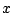is measured from the unstretched position of the spring.

2. Determine the equation of motion for the system ifis measured from the equilibrium position of the system, i.e.,is measured from the position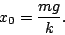Hint: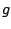should not appear in the answer for the second case.

6. In class we showed that the solution towith the appropriate definitions of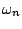and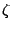was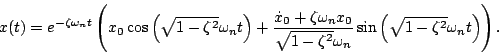Let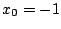and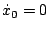.

On the same plot, plot the solution for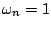and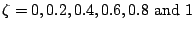for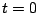to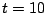.

Last updated: September 3, 2003.
B. Goodwine (goodwine@controls.ame.nd.edu)
J. Lucey (jlucey@nd.edu)